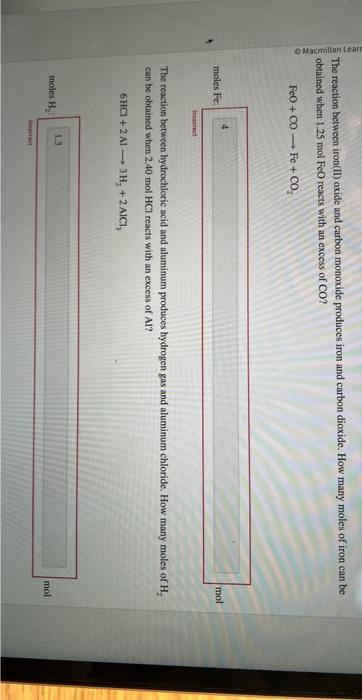Home / Expert Answers / Chemistry / how-would-you-solve-this-the-reaction-between-iron-ii-oxide-and-carbon-monoxide-produces-iron-and-pa208

# (Solved): how would you solve this? The reaction between iron(II) oxide and carbon monoxide produces iron and ...

how would you solve this?The reaction between iron(II) oxide and carbon monoxide produces iron and carbon dioxide. How many moles of iron can be obtained when $$1.25 \mathrm{~mol} \mathrm{FeO}$$ reacts with an excess of $$\mathrm{CO}$$ ? $\mathrm{FeO}+\mathrm{CO} \longrightarrow \mathrm{Fe}+\mathrm{CO}_{2}$ moles Fe incerrect The reaction between hydrochloric acid and aluminum produces hydrogen gas and aluminum chloride. How many moles of $$\mathrm{H}_{2}$$ can be obtained when $$2.40 \mathrm{~mol} \mathrm{HCl}$$ reacts with an excess of $$\mathrm{Al}$$ ? $6 \mathrm{HCl}+2 \mathrm{Al} \longrightarrow 3 \mathrm{H}_{2}+2 \mathrm{AlCl}_{3}$ moles H

We have an Answer from Expert